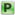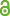Hauptmenü
• Autor
• Bazarova, Alina
• Berkes, István
• Raseta, Marko
• TitelOn trigonometric sums with random frequencies
• Datei
• LicenceCC BY
• Zugriffsrechte• AbstractWe prove that if $$I_k$$ are disjoint blocks of positive integers and $$n_k$$ are independent random variables with uniform distribution on $$I_k$$ , then $$N^{−1/2} Σ^N_{k=1} (sin 2πn_k x − \mathbb{E}(sin 2πn_k x))$$ has, with probability 1, a mixed Gaussian limit distribution relative to the interval (0, 1) equipped with Lebesgue measure. We also investigate the case when n k have continuous uniform distribution on disjoint intervals $$I_k$$ on the positive axis.